# Electric Potential

Electric potential at a point in an electric field is defined as the amount of work to be done to bring a unit positive electric charge from infinity to that point.

Similarly, the potential difference between two points is defined as the work required to be done for bringing a unit positive charge from one point to other point.
When a body is charged, it can attract an oppositely charged body and can repulse a similar charged body. That means, the charged body has ability of doing work. That ability of doing work of a charged body is defined as electrical potential of that body.

If two electrically charged bodies are connected by a conductor, the electrons starts flowing from lower potential body to higher potential body, that means current starts flowing from higher potential body to lower potential body depending upon the potential difference of the bodies and resistance of the connecting conductor.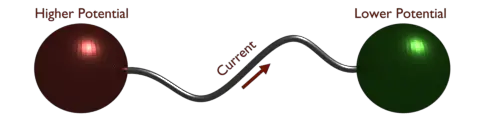So, electric potential of a body is its charged condition which determines whether it will take from or give up electric charge to other body.
Electric potential is graded as electrical level, and difference of two such levels, causes current to flow between them. This level must be measured from a reference zero level. The earth potential is taken as zero level. Electric potential above the earth potential is taken as positive potential and the electric potential below the earth potential is negative.

The unit of electric potential is volt. To bring a unit charge from one point to another, if one joule work is done, then the potential difference between the points is said to be one volt. So, we can say,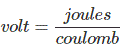If one point has electric potential 5 volt, then we can say to bring one coulomb charge from infinity to that point, 5 joule work has to be done.
If one point has potential 5 volt and another point has potential 8 volt, then 8 – 5 or 3 joules work to be done to move one coulomb from first point to second.

## Potential at a Point due to Point Charge

Let us take a positive charge + Q in the space. Let us imagine a point at a distance x from the said charge + Q. Now we place a unit positive charge at that point. As per Coulomb’s law, the unit positive charge will experience a force,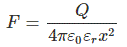Now, let us move this unit positive charge, by a small distance dx towards charge Q.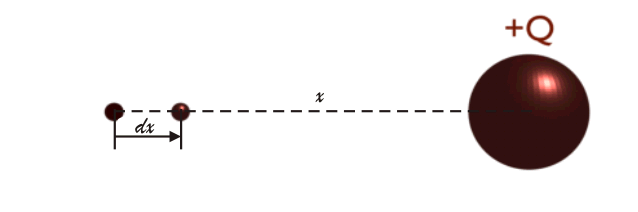During this movement the work done against the field is,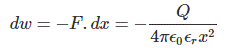So, total work to be done for bringing the positive unit charge from infinity to distance x, is given by,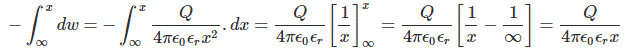As per definition, this is the electric potential of the point due to charge + Q. So, we can write,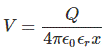## Potential Difference between Two Points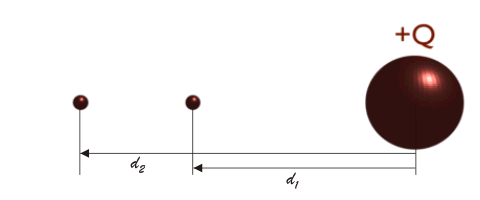Let us consider two points at distance d1meter and d2 meter from a charge +Q.
We can express the electric potential at the point d1 meter away from +Q, as,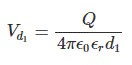We can express the electric potential at the point d2 meter away from +Q, as,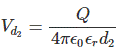Thus, the potential difference between these two points is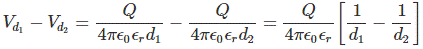Want To Learn Faster? 🎓
Get electrical articles delivered to your inbox every week.
No credit card required—it’s 100% free.